# TI-34 MultiView™Scientific Calculator

Intermediate, four-line scientific calculator with advanced fraction capabilities for middle school math and science.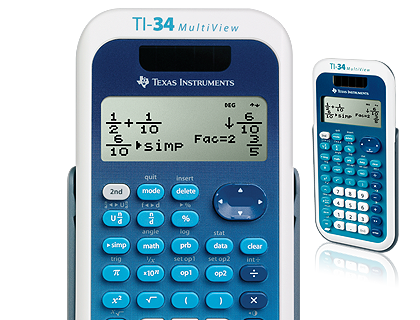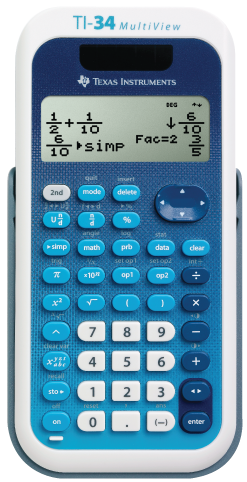## Key Features

• Four-line display
• One- and two-variable statistics
• MathPrint™ feature
• Step-by-step fraction simplification
• Edit, cut, and paste entries
• Solar and battery powered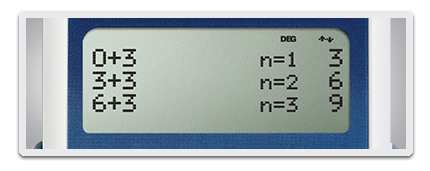## View more calculations at a time

Four-line display allows you to enter more than one calculation, compare results and explore patterns, all on the same screen.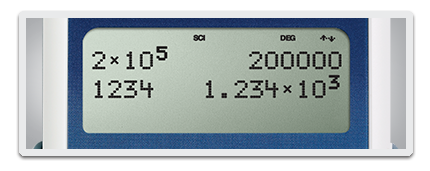## MathPrint™ feature

Use the MathPrint™ feature to display expressions, symbols, and fractions just as they appear in textbooks.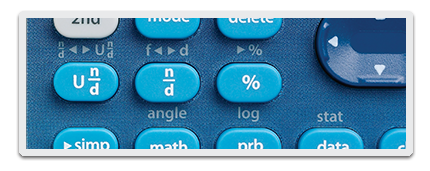## Explore fractions

Explore fraction simplification, integer division and constant operators.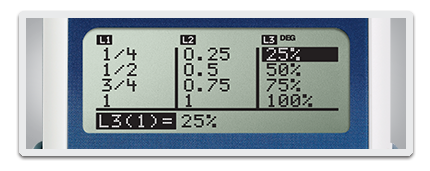## Investigate patterns

Explore patterns via list conversions to see different number formats like decimal, fraction and percent side-by-side.# TI-34 MultiView™ Scientific Calculator Specifications#### Built-in functionality

• Review and edit previous entries via a scrollable home screen
• Paste inputs or outputs into new calculations
• MathPrint™ mode for input in math notation, including π, square roots, fractions, percents and exponents plus math notation output for fractions
• CLASSIC mode for similar entry and compatibility with previous two-line scientific models like the TI-34II pixels
• π symbol on input, some calculations in terms of pi and pi to the maximum decimal places of the calculator
• Toggle key to change the form of answers between fraction and decimal, or between π terms and decimal
• Fraction/decimal/percent conversions
• Change between improper fractions and mixed numbers, plus a mode setting for mixed number or improper fraction output as the default
• Simplification of fractions feature to simplify a fraction one factor at a time instead of automatically simplifying to lowest terms
• Integer division denotes quotient and remainder
• Random number and random integer generator
• MODE menu for selecting calculator mode settings
• Functions accessed directly through keys or through vertical menus
• Negation key
• Two constant operation features for exploring mathematical patterns
• Combinations and permutations
• Trigonometry functions including sin, cos, tan, inverse sin, inverse cos and inverse tan
• Logs and antilogs
• Convert angles from degrees to radians
• Square root function is primary key
• %, x², ¹/x, π, x!, exponent key
• Fixed decimal mode option
• Basic Data/List Editor with three lists
• One- and two-variable statistics with stat variable input storage
• EOS: (Equation Operating System)
• Up to eight pending operations
• Up to 23 levels of parentheses
• Error recovery capability
• Quick/easy reset of calculator via 2 key press or menu for exam purposes
• Seven memory variables. (x,y,z,t,a,b,c)
• Scientific notation
• x10n key for quick input of numbers in powers of 10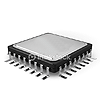#### Hardware

• Four-line × 16-character, easy-to-read LCD display
• Battery powered with solar cell assistance to lengthen battery life
• Auto Power Off
• Hard plastic, color-coded keys
• Non-skid rubber feet
• Impact-resistant cover with quick-reference card
• EZ-Spot Teacher Kit Available (includes 10 EZ Spot yellow calculators, storage caddy, Teacher's Guide in English and Spanish, calculator poster and transparency)#### Support## TI-34 MultiView™ Scientific Calculator Accessories

Extend the power of your calculator with accessories for the TI-34 MultiView™ scientific calculator.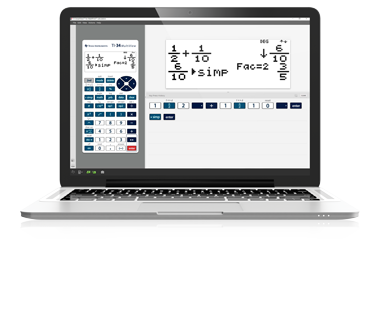## TI-SmartView™ emulator for MathPrint™ calculators

This simple software complements the TI-34 MultiView™ scientific calculator, letting the educator project a representation of the calculator’s display to the entire class. It is an ideal demonstration tool for leading classroom instruction of math and science concepts.